cancel
Showing results for
Did you mean:Helper III

## Calculate the value closest to selected date range

Hi,

to simplify my question, I have a table like this. The column Quantity represents the change of stock on given date and column Current stock represents the value I want to find.and a standard calendar table - 'Calendar'[Dates] with unique dates.

I need an outcome like this: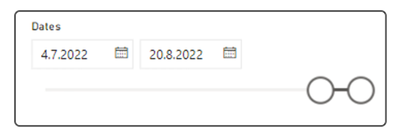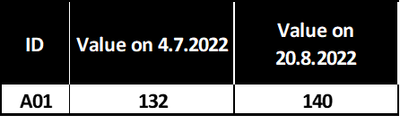The logic is that the formula has to find the nearest date lower or equal to the min and max range selection, so in this case dates 1.7.2022 and 19.8.2022. There are obviously many other IDs and Warehouses involved as well as the 'StockTable'[Date] has duplicate values. The best I could do is something like this for the upper part of the range, but it does not work. Can you please help me?

``````Latest date stock =
var selectedmaxdate = MAX('Calendar'[Dates])
return
CALCULATE(
SUM(StockTable[Current stock]),
'Calendar'[Dates] <= selectedmaxdate,
LASTDATE('Calendar'[Dates])
)``````
1 ACCEPTED SOLUTIONSuper User

See if this works for you.

(I've added a Dimension for Warehouse to the model)

Measure for the stock at min date selected:

``````Stock at Min Selection =
VAR _MinSel =
MIN ( 'Calendar'[Dates] )
VAR _Stock =
LASTNONBLANKVALUE (
FILTER ( ALL ( 'Calendar'[Dates] ), 'Calendar'[Dates] <= _MinSel ),
[Sum Stock]
)
RETURN
_Stock
``````

Measure for the stock at max date selected:

``````Stock at Max Selection =
VAR _MaxSel =
MAX ( 'Calendar'[Dates] )
VAR _Stock =
LASTNONBLANKVALUE (
FILTER ( ALL ( 'Calendar'[Dates] ), 'Calendar'[Dates] <= _MaxSel ),
[Sum Stock]
)
RETURN
_Stock
``````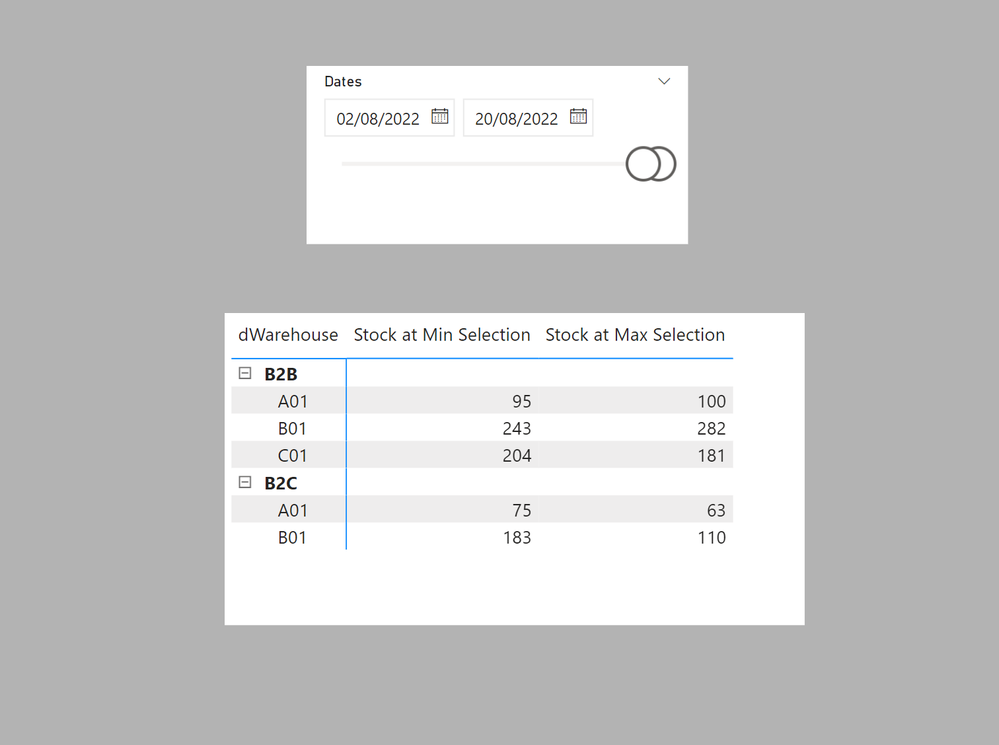I've attached the sample PBIX file

In doing so, you are also helping me. Thank you!

Proud to be a Super User!

20 REPLIES 20Super User

See if this works for you.

(I've added a Dimension for Warehouse to the model)

Measure for the stock at min date selected:

``````Stock at Min Selection =
VAR _MinSel =
MIN ( 'Calendar'[Dates] )
VAR _Stock =
LASTNONBLANKVALUE (
FILTER ( ALL ( 'Calendar'[Dates] ), 'Calendar'[Dates] <= _MinSel ),
[Sum Stock]
)
RETURN
_Stock
``````

Measure for the stock at max date selected:

``````Stock at Max Selection =
VAR _MaxSel =
MAX ( 'Calendar'[Dates] )
VAR _Stock =
LASTNONBLANKVALUE (
FILTER ( ALL ( 'Calendar'[Dates] ), 'Calendar'[Dates] <= _MaxSel ),
[Sum Stock]
)
RETURN
_Stock
``````I've attached the sample PBIX file

In doing so, you are also helping me. Thank you!

Proud to be a Super User!Helper III

Hi @PaulDBrown , thank you very much for this. It works great, however it does not reflect one thing. As I mentioned in the original post, the StockTable[Date] column has duplicates even for the same IDs and Warehouses meaning more than one stock movement for one product in a day is possible.

See for example 21.6.2022 for the product B01. Correct value to be shown is the last stock movement = 173 pcs. I did not include times in the sample file so we cannot say what the last movement is. I did not think of it.

However, if there was time included in the Date column, would it show the last value in that day only and not the sum of all the values with selected date?

And is there any way to show only one value of Current stock if there are more records in one day without a time value?

For example as you can see below, there are three records on June 21st, 2022, but the measure would take only one (it does not matter which one) and show 173 pcs (or 170 or 199, really doesn't matter, but not the sum of it)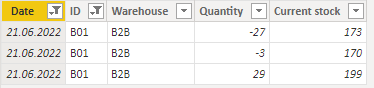Super User

Does your original data contain a Date/time field then?

In doing so, you are also helping me. Thank you!

Proud to be a Super User!Helper III

Yes, but not in an ideal way. It sometimes does contain the time data, sometimes not. It depends on the person entering the data and I have no control over it. It looks like this: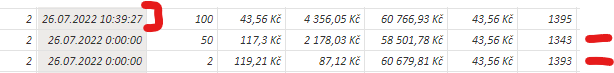Anyway, you've been an incredible help to me already, so thank you.

I would like to have one last question: If the data is like the picture above for the specific day, the Sum Stock shows the sum of only those rows without the time value.

I was wondering that using AVERAGE instead of SUM would be enough for my purpose, which is to show only one value for the day if there are more records.

BUT as you are surely well aware of it does not show the data for the day if there is only one record for specific day which includes the time value like this:If I choose 10.9.2022, it shows value 4 and not 24, which it should show.

Can this issue (mix of date and date/time) be solved?

EDIT:

Converting the date column to date column only in query editor and then using average instead of sum for the value for specific day (if more than one record for the day is present) basicaly solved those issues. I marked your original reply as a solution as this is just working great 🙂 Thank you again.

Your potential suggestions would be greatly appreciated though 🙂Super User

Assuming that,  even though the date/time field is inconsistent, the order of the rows reflects the chronological integrity, you could add an index column to the table. In that case, the highest index on a particular day would be showing the "closing" day's stock.

In doing so, you are also helping me. Thank you!

Proud to be a Super User!Helper III

Hi @PaulDBrown  again. I discovered a problem. You can see it in attached file.

Problem is that dimensions warehouse and product do not show the same numbers. I think I know why but I don't know the solution.As you can see, there are 63 pcs of product A01 and 110 pcs of product B01 at the end date, but the warehouse only shows 63 pcs. I suppose product A01 is the last non blank cell and the formula just takes the nearest date of one product. So it ignores other products when trying to show the warehouse numbers.

Can this be solved?

It is correctly shown in the Matrix table you created but only if products are expanded. I want to be able to see the summarized number for warehouses (without seeing thousands of rows of product as in the original data there are thousans of products).Super User

If you remove the product field, the LASTNONBLANKVALUE  will be for the Warehouse value only; the measures depend on the filter context.

I did not include the total calculation in the original suggestion since I assumed that adding units of random products made no sense (cars + wardrobes...). However, it's easy to solve using SUMX, and follwoing this pattern:

``````Total Stock at Min Sel =
SUMX(Products, [Stock at Min Selection])``````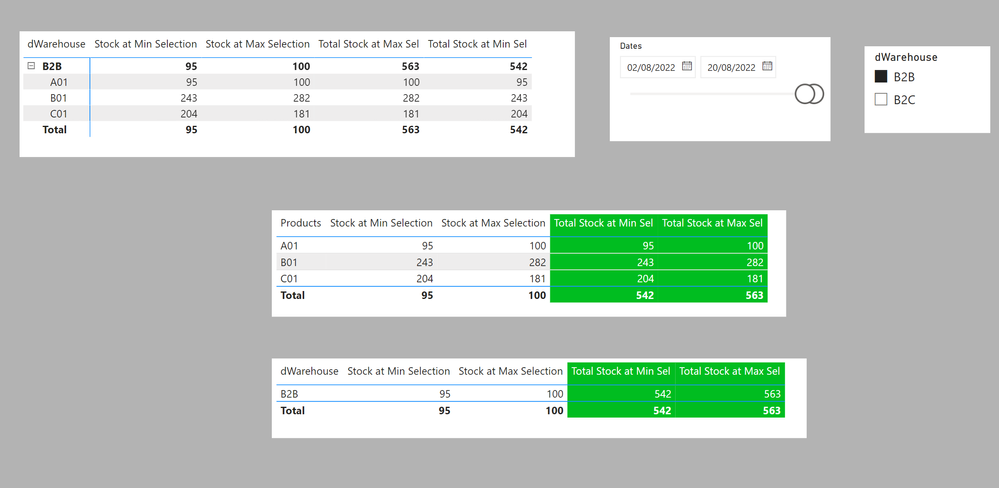If you would like to solve the SUM issues you mentioned earlier, I have included a solution using the Index field which I added to the StockTable. The measures follow these patterns:

``````INDEX Stock at Min Selection =
VAR _MinSel =
MIN ( 'Calendar'[Dates] )
VAR _MXIndex =
CALCULATE (
MAX ( StockTable[Index] ),
FILTER (
ALL ( 'Calendar'[Dates] ),
'Calendar'[Dates] <= _MinSel
&& NOT ( ISBLANK ( [Sum Stock] ) )
),
ALLEXCEPT ( StockTable, Products[Products], 'Dim Warehouse'[dWarehouse] )
)
RETURN
CALCULATE (
[Sum Stock],
FILTER ( ALL ( StockTable ), StockTable[Index] = _MXIndex )
)
``````
``````INDEX Total Stock at Min Sel =
SUMX(Products, [INDEX Stock at Min Selection])``````I've attached the sample PBIX file

In doing so, you are also helping me. Thank you!

Proud to be a Super User!Helper III

Hi @PaulDBrown ,

I hope I don't bother you with my questions already. Since the time you helped me I implemented those solutions and learned a lot from it.

However, when using this model I have run into a problem. In the sample file and also in the picture you posted, there is 563 pcs total stock at max date in B2B warehouse and 181 pcs in B2C warehouse. That is perfectly fine, but I did not find the way to show total stock across both warehouses. Is it possible without completely rewriting the code get a total value too?

For example if I have a simple table showing just products no matter the warehouse.

Value for all the products should be 744 pcs

OR

Product A01 ... 163 pcs

Product B01 ... 400 pcs

Product C01 ... 181 pcs

I hope this filter context is not completely lost, I don't want to bother you with rewriting the code, just a little tweak would be great.Super User

See if these new measures work:

``````Totals at MIN =
SUMX (
SUMMARIZE ( StockTable, Products[Products], 'Dim Warehouse'[dWarehouse] ),
[INDEX Stock at Min Selection]
)
``````
``````Totals at MAX =
SUMX (
SUMMARIZE ( StockTable, Products[Products], 'Dim Warehouse'[dWarehouse] ),
[INDEX Stock at Max Selection]
)
``````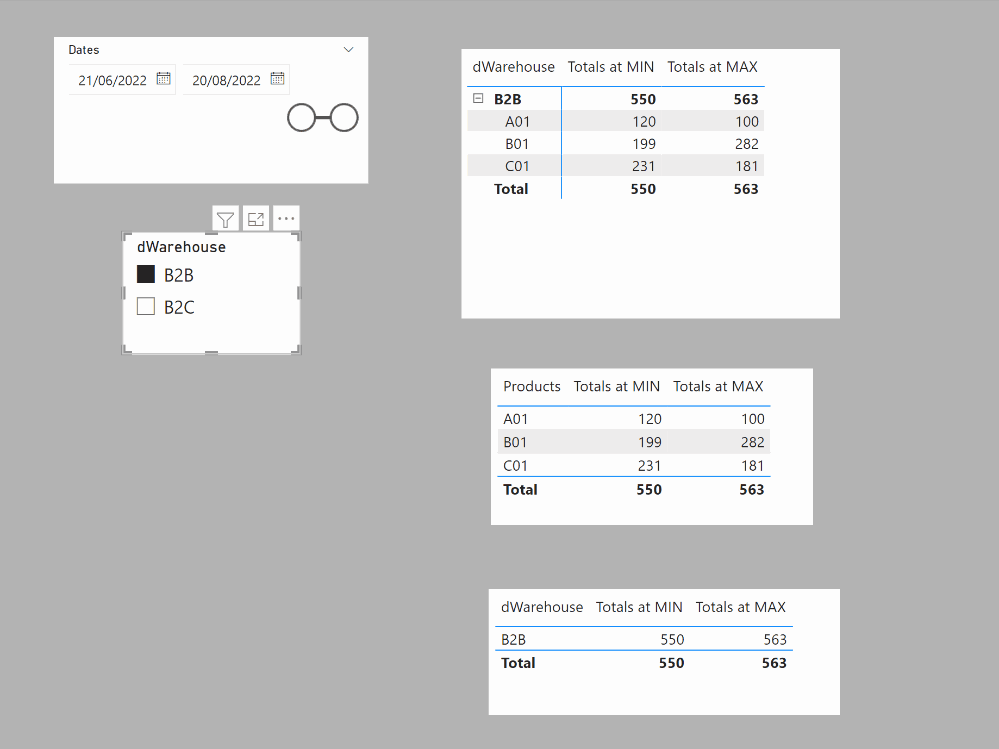In doing so, you are also helping me. Thank you!

Proud to be a Super User!Helper III

Hi @PaulDBrown , it's me again 🙄.

I found an error that contradicts the logic of the report. New measures work great for the purpose of showing total stock across both warehouses. However, I found out that the min value (start date) of the date slicer affects the max value (end date) in some cases.

Max value though should be independent of the min value date slicer, because it should be showing the stock value on the selected max date no matter the start date. But it is not.

I added new measures in this sample file and the total stock value on the end date is almost always 744 pcs, which is right. But if I move the start date closer to the end date, the end date starts to get affected.

For example if the start date is 16.8.2022 (with end date being always 20.8.2022), then the end date stock value decreases to 445 pcs.

I get that it has something to do with using summarize, but I just don't know how to remove the start date parameter out of the measure calculation.

Is it even possible to fix this and still use the SUMMARIZE function?Super User

Yes, I see what you mean. The measure is not actually returning the Index needed, since it appears that the ALL() is negating the subsequent date reference. My apologies since I should have spotted that!

I've tested the following to calculate the following which return the correct stock values at max and min date references:

``````INDEX Stock at Min Selection =
VAR _MinSel =
MIN ( 'Calendar'[Dates] )
VAR _MXIndex =
CALCULATE (
MAX ( StockTable[Index] ),
FILTER ( ALL ( StockTable[Date] ), StockTable[Date] <= _MinSel ),
ALLEXCEPT ( StockTable, Products[Products], 'Dim Warehouse'[dWarehouse] )
)
RETURN
CALCULATE (
[Sum Stock],
FILTER ( ALL ( StockTable ), StockTable[Index] = _MXIndex )
)
``````

``````INDEX Stock at Max Selection =
VAR _MinSel =
CALCULATE ( MAX ( 'Calendar'[Dates] ), ALLSELECTED ( 'Calendar'[Dates] ) )
VAR _MXIndex =
CALCULATE (
MAX ( 'StockTable'[Index] ),
FILTER ( ALL ( StockTable[Date] ), StockTable[Date] <= _MinSel ),
ALLEXCEPT ( StockTable, Products[Products], 'Dim Warehouse'[dWarehouse] )
)
RETURN
CALCULATE (
[Sum Stock],
FILTER ( ALL ( StockTable ), StockTable[Index] = _MXIndex )
)
``````

Keeping the final SUMX measures as you have them (just beware that the correct totals are with SUMX(SUMMARIZE())...)New file attached

In doing so, you are also helping me. Thank you!

Proud to be a Super User!Helper III

I can see how the numbers are now correct (total sum is 736 instead of 744) but there is still one bug. If I choose the start date - end date like the first picture (27.7.2022 - 20.8.2022), values are start date = 732 and end date = 736.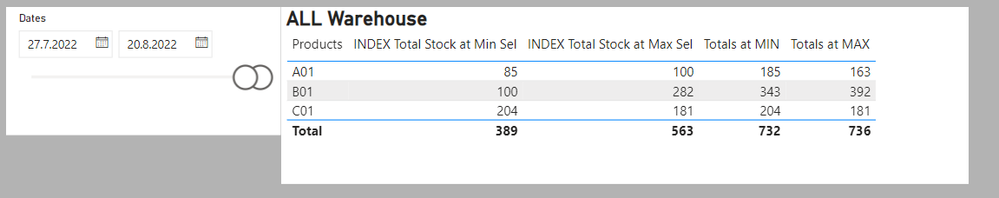But if I move the start date close to end date, the end date value (max value) changes to

end date = 445.Since the end date value should always be the same (the value of stock on 20.8.2022) no matter the start date, it is still not working.

In other words, no matter the min date selected in the date slicer, the max date value should always be the same. I am simply asking a question: What was the stock value on 20.8.2022 regardless of what start date I choose?

Does my question make sense? I hope you understand

Should I maybe use two separate date slicers, one for the min value and one for the max value? I just want to define two days (two point in time) that I want to compare without any interest for what happened "between" those two days.Super User

Well this is turning out to be quite a challenge. Sorry about the "measure testing"...

I think (HOPE!) this structure will sort things out and provide final measures...

``````Totals at MIN =
SUMX (
VALUES ( 'Dim Warehouse'[dWarehouse] ),
SUMX ( Products, [INDEX Stock at Min Selection] )
)
``````
``````Totals at MAX =
SUMX (
VALUES ( 'Dim Warehouse'[dWarehouse] ),
SUMX ( Products, [INDEX Stock at Max Selection] )
)
``````Nested SUMX are not a good idea, but I haven't been able to find another working solution unfortunately.

New file attached

In doing so, you are also helping me. Thank you!

Proud to be a Super User!Helper III

Paul, please don't apologize for anything, you have been an incredible help. This does exactly what I want.

However in my original model with data I cannot share (that is why I created a simplified model) there are 4 other subcategories of Product. For Example product A01 is Accessories, Product B is Bikes etc. The previous measures worked for the subcategories as well so I did not mention it. But with these last measures it seems like I lost a filter context for anything else than Product and Warehouse.

I added a subcategory Type into the model like this:and the filter context is lost:I am really sorry I keep adding to the problem, but I as mentioned these are the first measures that do not work for the subcategories. Only work for product and warehouse.

EDIT: Oh, seems like adding those dimensions (subcategories) to the ALLEXCEPT statement of the index measures solves the problem. I don't want to waste your time when not necessary, so can you just please confirm that this is OK?

I really respect your time but I got this idea just right after posting the original reply 🙂Super User

Yes, you need to include all the fields over which the index reference is to be calculated in the ALLEXCEPT expression

In doing so, you are also helping me. Thank you!

Proud to be a Super User!Helper III

I have the utmost respect for you @PaulDBrown , works exactly like it is supposed to. Thank you!Helper III

@PaulDBrown  thank you so much for your help in the previous days. Works as it is supposed to!

I don't want to steal your time anymore, but is there an article or something about how this trick with index column works? Your code is obviously great but I don't understand it 🙂Super User

No problem. See if I can clarify the method. I am assuming that the dataset is constructed chronologically and that the integrity is kept on import. This means that each row moves forward chronologically. When you add an index column, it reflects the chronological nature (so row 3 is "newer" than row 2 which is "newer" than row 1...So if you then have more than 1 row for any particular date, the highest index will be the more recent set of data. Make sense?

As for the measure...

``````INDEX Stock at Min Selection =
VAR _MinSel =
MIN ( 'Calendar'[Dates] )
VAR _MXIndex =
CALCULATE (
MAX ( StockTable[Index] ),
FILTER (
ALL ( 'Calendar'[Dates] ),
'Calendar'[Dates] <= _MinSel
&& NOT ( ISBLANK ( [Sum Stock] ) )
),
ALLEXCEPT ( StockTable, Products[Products], 'Dim Warehouse'[dWarehouse] )
)
RETURN
CALCULATE (
[Sum Stock],
FILTER ( ALL ( StockTable ), StockTable[Index] = _MXIndex )
)``````

We need the MAX index value for each Warehouse/Product before or on the minimum (in this example) date selected.

The first filter creates a table fo dates which are less than or equal to the minimum date selected. (you need the ALL to remove the filter in the selection, or you would not get dates before the selection).

The second filter with ALLEXCEPT removes the filters on the StockTable except for rows affected by the columns specified in the expression (so Warehouse and Product are kept). You can read about how this expression works in this video by the gurus at sqlbi.com

The final code in the RETURN  produces the sum of the stock value for the rows where the index is equal to the calculated max index by warehouse and product.

To get the sum of values, you need to calculate the stock value by product and then sum these values up. This is where SUMX comes in. SUMX iterates over the product table calculating the stock value by product and then sums these values up.

A shot video on SUMX:

Hope that helps!

In doing so, you are also helping me. Thank you!

Proud to be a Super User!Super User

Can you please provide sample data or a PBIX file?

In doing so, you are also helping me. Thank you!

Proud to be a Super User!Helper III

Yes of course, thank you for participating in this thread.

Here is the file: Sample file

Correct numbers would be, lets say for product A01:

stock at 2.8.2022 = 95 for B2B warehouse and 75 for B2C

stock at 20.8.2022 = 100 for B2B warehouse and 63 for B2CAnnouncements#### Exclusive opportunity for Women!

Join us for a free, hands-on Microsoft workshop led by women trainers for women where you will learn how to build a Dashboard in a Day!#### Power Platform Conference-Power BI and Fabric Sessions

Join us Oct 1 - 6 in Las Vegas for the Microsoft Power Platform Conference.Top Solution Authors
Top Kudoed Authors
Users online (1,589)